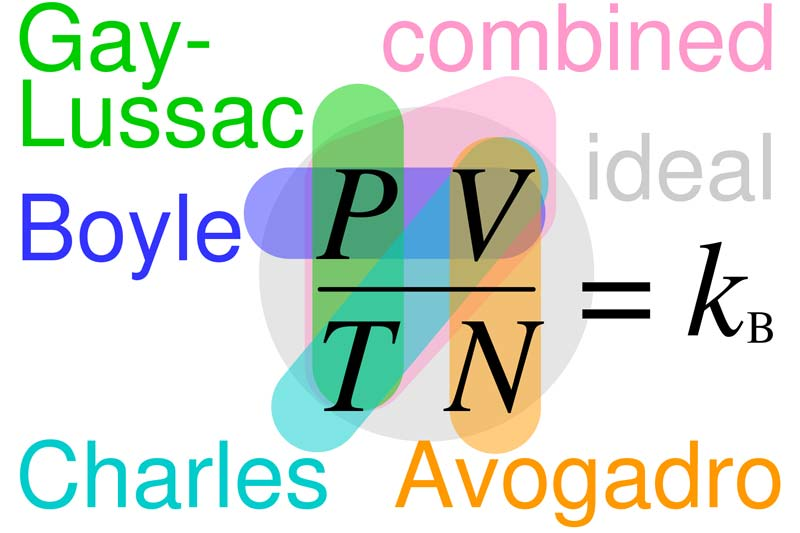A# gas lawsRelationships between Boyle's, Charles's, Gay-Lussac's, Avogadro's, combined, and ideal gas laws, with the Boltzmann constant k B = R/NA = n R/N. In each law, properties circled are variable and properties not circled are held constant.

The gas laws are statements concerning the volume change of gases under the effect of alterations of pressure and temperature.

Boyle's law states that at constant temperature the volume of a given mass of gas is inversely proportional to the pressure, i.e. pV = constant.

Charles' law states that at constant pressure all gases expand by 1/273 of their volume at 0°C for a rise in temperature of 1°C; i.e. the volume of a given mass of gas at constant pressure is directly proportional to the absolute temperature.

The two laws may be combined in the expression pV is proportional to T, where T is the absolute temperature; or, for a gram-molecule of a gas, pV = RT. This gives the behavior of a gas when both temperature and pressure are altered.

The gas laws are not perfectly obeyed by ordinary gases, being strictly true only for an ideal gas. Various equations have been derived which attempt to give a better approximation to the behavior of actual gases. The best known of these is van der Waals' equation of state.